# Quiz 16: Risk Analysis

Business

Risk averse investors are the ones who aim to minimize the risk. Thus risk averse investors demand for higher risk premium to adjust for the high risk involved in the investment projects. Risk averse investors have a low expectation of a higher payoff and generally expect a constant payoff with a minimum standard deviation. Thus the risk averse investors prefer lower as opposed to higher coefficients of variation. Risk neutral investors place a maximum value on a project exactly equal to their expected rewards. Therefore, certain sums and expected risky sums of equal dollar amounts are valued equally by risk neutral investors. Risk-seeking investors are the ones with increasing marginal utility of money, because increase in their wealth increases the utility of that wealth more than proportionately and thus they are aggressive investors. Risk neutral investors place a value on project exactly equal to the expected returns, irrespective of variability in returns due to risk involved.

Economic risk refers to the possibility of loss when all the potential outcomes and their probabilities are unknown. Actions taken under the environment of economic risk are based on speculations. For example, trading of derivative instruments such as options and futures involves speculations of future prices. In contrast, uncertainties occur when outcomes of managerial decisions cannot be predicted with accuracy however, the probabilities associated with the outcomes are known. Similar to economic risk, in the case of uncertainties also luck plays a major role in determining success, but experience and insights to devise strategies can bring down the probability of failure unlike in the case of economic risks.

The certainty equivalent adjustment factor a indicates the maximum price in certain dollars that an individual is willing to pay per risky dollar of expected return. Thus, the statement is false. The statement is true. Certainty equivalent adjustment factor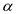is the ratio of certain sum to expected risky return. So when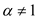the certain sum and expected risky return would differ. Certainty equivalent adjustment factor a can be given as,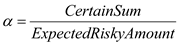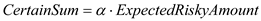…… (1) Expected risky return from the project is \$150,000. Therefore, when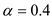, equivalent certain sum or cost will be,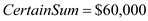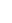When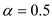, equivalent certain sum or cost will be,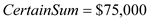The maximum certain sum equivalent of an expected risky \$150,000 falls in the range between \$60,000 and \$75,000. Hence the above statement is true as \$50,000 is less than the lower limit of the range i.e. \$60,000. The statement is false. A project that has NPV 0 using the risk adjusted discount rate approach has anfactor below the maximum acceptable level. Such a project will be desirable, because it has a dollar price per risky dollar of return below that necessary to induce investment. The statement is true. For state lotteries that pay out 50% of the revenues that they generate require players with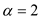.